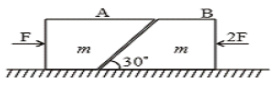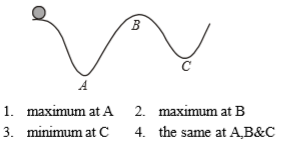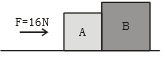Two blocks 'A' and 'B' each of mass 'm' are placed on a smooth horizontal surface. Two horizontal force F and 2F are applied on both the blocks 'A' and 'B' respectively as shown in figure. The block A does not slide on block B. Then the normal reaction acting between the two blocks is:(1) F

(2) F/2

(3) $\frac{F}{\sqrt{3}}$

(4) 3F

Concept Questions :-

Application of laws
High Yielding Test Series + Question Bank - NEET 2020

Difficulty Level:

Five persons A, B, C, D & E are pulling a cart of mass 100 kg on a smooth surface and the cart is moving with acceleration 3 $\mathrm{m}/{\mathrm{s}}^{2}$ in east direction. When person 'A' stops pulling, it moves with acceleration 1 $\mathrm{m}/{\mathrm{s}}^{2}$ in the west direction. When person 'B' stops pulling, it moves with acceleration 24 $\mathrm{m}/{\mathrm{s}}^{2}$ in the north direction. The magnitude of the acceleration of the cart when only A & B pull the cart keeping their directions same as the old directions are:

1.  26 $\mathrm{m}/{\mathrm{s}}^{2}$

2.

3.  25 $\mathrm{m}/{\mathrm{s}}^{2}$

4.  30 $\mathrm{m}/{\mathrm{s}}^{2}$

Concept Questions :-

Application of laws
High Yielding Test Series + Question Bank - NEET 2020

Difficulty Level:

A body moves along an uneven surface with constant speed at all points.

The normal reaction due to ground on the body is :Concept Questions :-

Uniform circular motion
High Yielding Test Series + Question Bank - NEET 2020

Difficulty Level:

The forces required to just move a body up an inclined plane is double the force required to just prevent it from sliding down. If $\mathrm{\varphi }$ is angle of friction and $\mathrm{\theta }$ is the angle which makes with horizontal, then

1.

2.

3.

4.

Concept Questions :-

Friction
High Yielding Test Series + Question Bank - NEET 2020

Difficulty Level:

If no external force acts on the system, then the correct statement is:

1.  velocity of centre of mass changes

2.  momentum of centre of mass is conserved and constant

3.  position of the center of mass changes with time

4.  None of these

Concept Questions :-

Newton laws
High Yielding Test Series + Question Bank - NEET 2020

Difficulty Level:

Two masses m and M are connected by a light string passing over a smooth pulley. When mass m moves up by 1.4 m in 2 sec, the ratio $\frac{m}{M}$ is

(1) $\frac{13}{15}$

(2) $\frac{15}{13}$

(3) $\frac{9}{7}$

(4) $\frac{7}{9}$

Concept Questions :-

Application of laws
High Yielding Test Series + Question Bank - NEET 2020

Difficulty Level:

Two blocks A and B of masses 4 kg and 12 kg are placed on a smooth plane surface. A force F of 16 N is applied on A as shown. The force of contact is :1. 4 N

2. 8 N

3. 12 N

4. 16 N

Concept Questions :-

Newton laws
High Yielding Test Series + Question Bank - NEET 2020

Difficulty Level:

The engine of a car produces acceleration 4 m/s2 in the car. If this car pulls another car of same mass, what will be the acceleration produced

(1) 8 m/s2

(2) 2 m/s2

(3) 4 m/s2

(4) $\frac{1}{2}m/{s}^{2}$

Concept Questions :-

Newton laws
High Yielding Test Series + Question Bank - NEET 2020

Difficulty Level:

A truck and a car are moving with equal velocity. If equal retarding force is applied on each, then on applying the brakes both will stop after certain distance

(1) Truck will cover less distance before rest

(2) Car will cover less distance before rest

(3) Both will cover equal distance

(4) None

Concept Questions :-

Newton laws
High Yielding Test Series + Question Bank - NEET 2020

Difficulty Level:

A constant force acts on a body of mass 0.9 kg at rest for 10s. If the body moves a distance of 250 m, the magnitude of the force is

(1) 3 N

(2) 3.5 N

(3) 4.0 N

(4) 4.5 N

Concept Questions :-

Newton laws